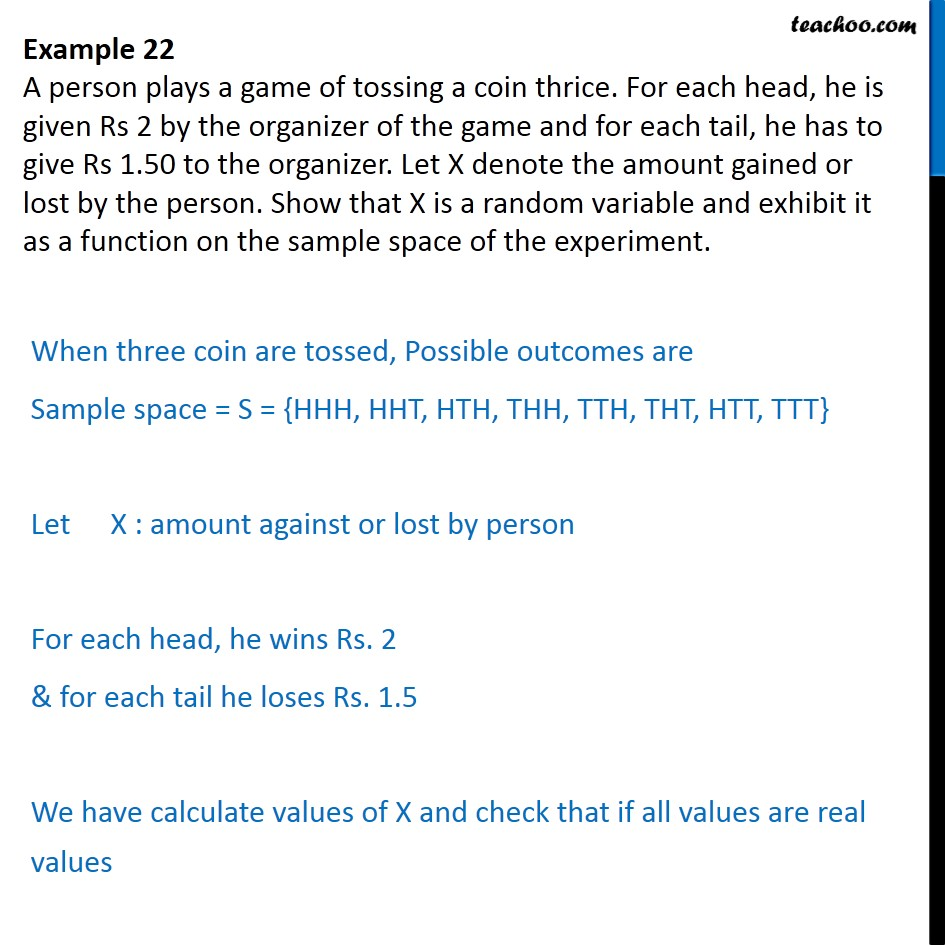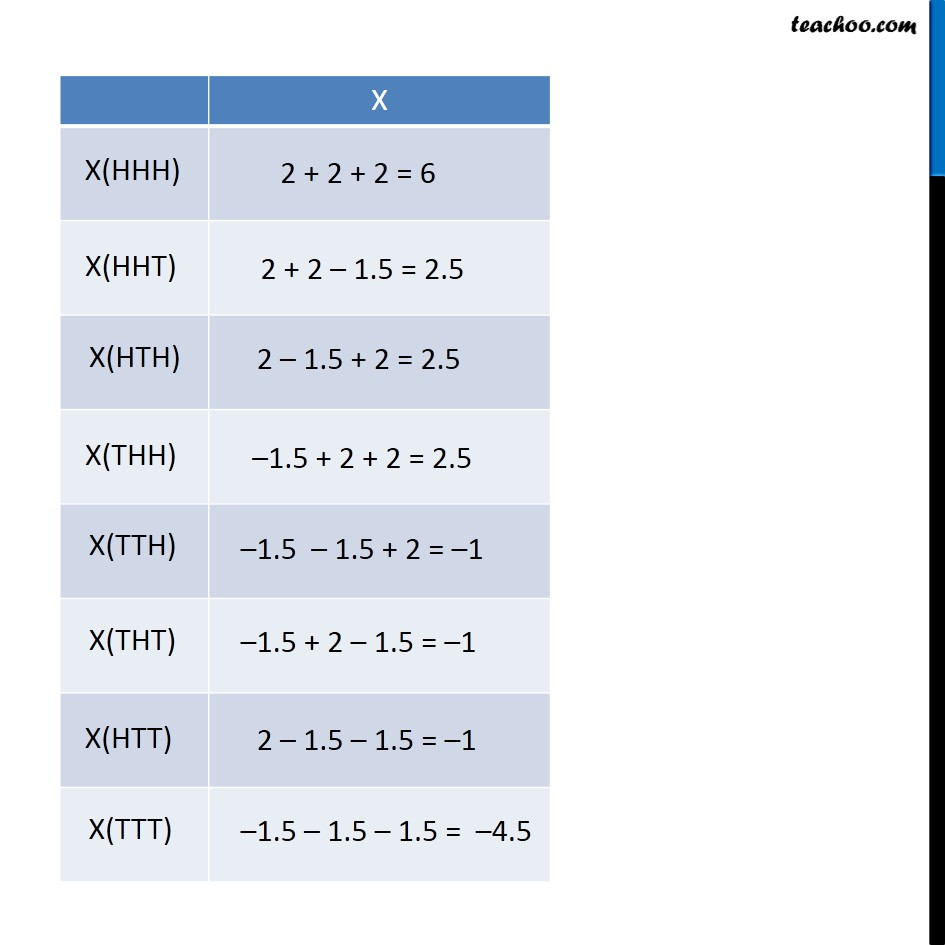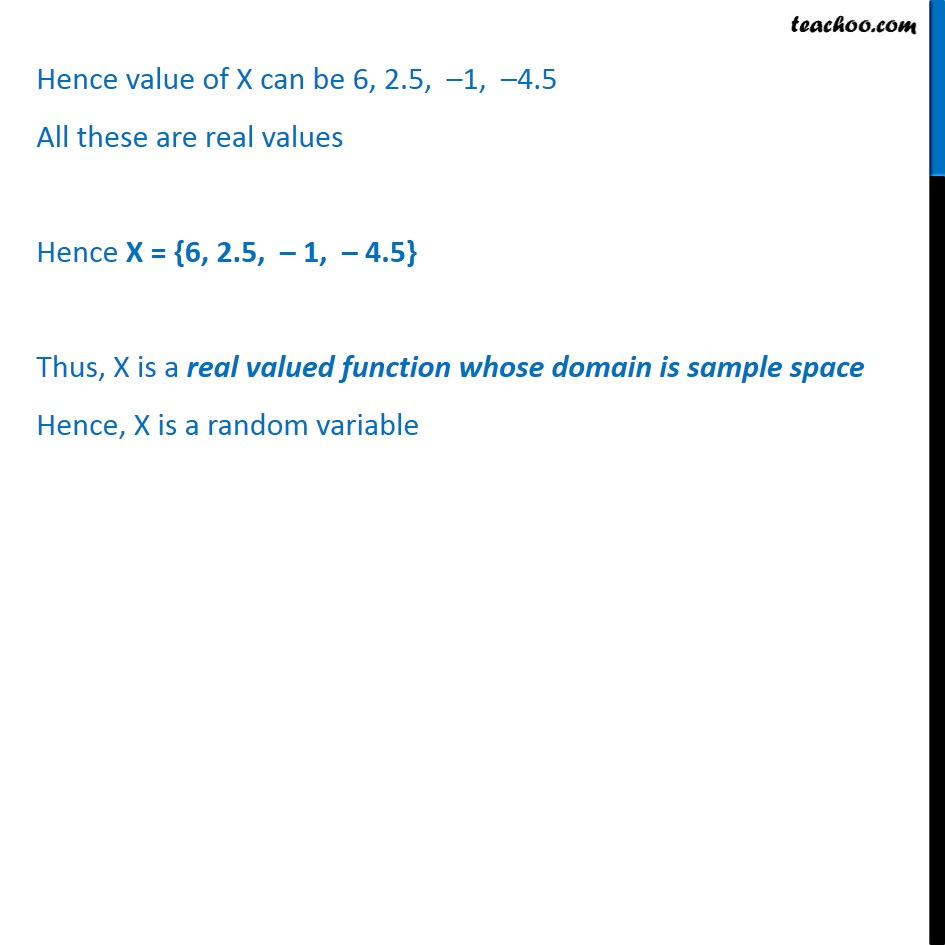1. Chapter 13 Class 12 Probability
2. Serial order wise
3. Examples

Transcript

Example 22 A person plays a game of tossing a coin thrice. For each head, he is given Rs 2 by the organizer of the game and for each tail, he has to give Rs 1.50 to the organizer. Let X denote the amount gained or lost by the person. Show that X is a random variable and exhibit it as a function on the sample space of the experiment. When three coin are tossed, Possible outcomes are Sample space = S = {HHH, HHT, HTH, THH, TTH, THT, HTT, TTT} Let X : amount against or lost by person For each head, he wins Rs. 2 & for each tail he loses Rs. 1.5 We have calculate values of X and check that if all values are real values Hence value of X can be 6, 2.5, 1, 4.5 All these are real values Hence X = {6, 2.5, 1, 4.5} Thus, X is a real valued function whose domain is sample space Hence, X is a random variable

Examples